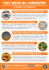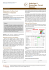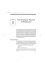# 9.1 Financial Mathematics: Borrowing Money

## Transcription

9.1 Financial Mathematics: Borrowing Money
```Math 3201
9.1 Financial Mathematics: Borrowing Money
Simple vs. Compound Interest
Simple Interest: the amount of interest that you pay on a loan is calculated ONLY based on
the amount of money that you borrow. That is, you only pay interest on
the money that you borrow.
Compound Interest: interest is paid on two different things:
(i) the amount of money that you borrow and
(ii) the interest that you pay on the money that you borrowed.
When we talk about borrowing money, there are two groups of people involved:
1. The customer is the person/group who borrows the money.
2. The lender is the the person/institution who loans out the money. It may be a
friend, a family member, bank, etc.
Different groups benefit differently from various types of interest. For example, a
compound rate involves a greater amount of interest being paid than a simple rate. The
lender, or person/group who loans out money would benefit more from this, since they will
earn more money in interest. The customer, or person/group who borrows the money is
disadvantaged by this however because it means they will pay out more in interest.
There are two main factors that affect the advantages and disadvantages of different types
of interest:
1. Whether you are a customer or lender: ie. compound interest is an advantage for a
2. Whether you are borrowing or investing money: simple interest would be better for a
customer who is borrowing money since it results in the customer having to pay out
less money in interest. However, compound interest is better for a customer who is
investing money since it means they will gain more money on their investment through
interest.
When are simple and compound interest rates typically used?
Simple Interest:
 loans from family members or friends
 loans or investments of a year or less
Compound Interest:
 Most products offered by financial institutions. See table below.
*Exception: GICs and Canada Savings Bonds can have either simple or compound interest.
Calculations Involving Simple and Compound Interest
Simple Interest Formula
𝐴 = 𝑃 + 𝑃𝑟𝑡
where
 A represents the amount present
 P represents the principal amount
 r = interest percentage divided by 100
 t represents the number of years
We can factor the P out of the right hand side of the equation to give:
𝐴 = 𝑃(1 + 𝑟𝑡)
Compound Interest Formula:
𝐴 = 𝑃(1 + 𝑖)𝑛
where
 P is the the principle amount
 𝑖 is the interest rate per compounding period
 𝑛 is the number of compounding periods
Notice that 𝑖 is the interest rate per compounding period. If interest is compounded 𝑥
times each year, then the given percentage must be divided by 𝑥 to come up with 𝑖.
Compounding periods are usually daily, weekly, semimonthly, monthly, quarterly,
semiannually or annually. The table below shows how many times interest is paid, and the
interest rate for each of these options.
Compound interest increases
linearly over time.
Simple interest increases
exponentially over time.
Both types graphed on
the same axis.
Example 1:
James intends to go to university in five years. His grandmother decides to invest \$2000 in
a Guaranteed Investment Certificate (GIC) to help with his first-year expenses.
(A) How much would the GIC be worth in 5 years if she chooses a simple interest GIC at
3% annual interest?
(B) How much would it be worth if the interest is compounded monthly?
(C) Which option is better for the bank?
(D) Which is better for James?
Example 2:
Suppose Peter borrows \$1000 from his parents.
(A) How much will he have to pay back in 2 years if they charge 3% simple interest per
year?
(B) How much will you have to pay back in 2 years if they charge 3% interest
compounded monthly?
(C) Explain which is the better option for him.
Example 3:
Predict which of the following investments would yield the greater return:
Option 1: \$1000 at 3.5% annual simple interest
Option 2: \$1000 at 3% annual compound interest
Verify your prediction by calculating the value of the investments after 5 years.
```

### Completing Chemical Reactions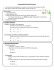### Compound V\lords Compound VVords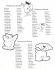### Simple and Compound Sentences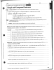### 7.5 Word Problems Involving Logarithms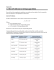### Untitled - IISER Pune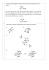### 1. A problem is listed below. Identify its type.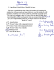### Rukan Brochure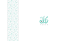### Modifying the Compound Rest on the 7x10 Mini Lathe For Quick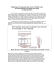### Organic Cumulative Exam February 13, 2014 LPSC 239 and LPSC 259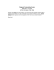### THIS WEEK IN CHEMISTRY 19 OCTOBER - 25 OCTOBER 2014